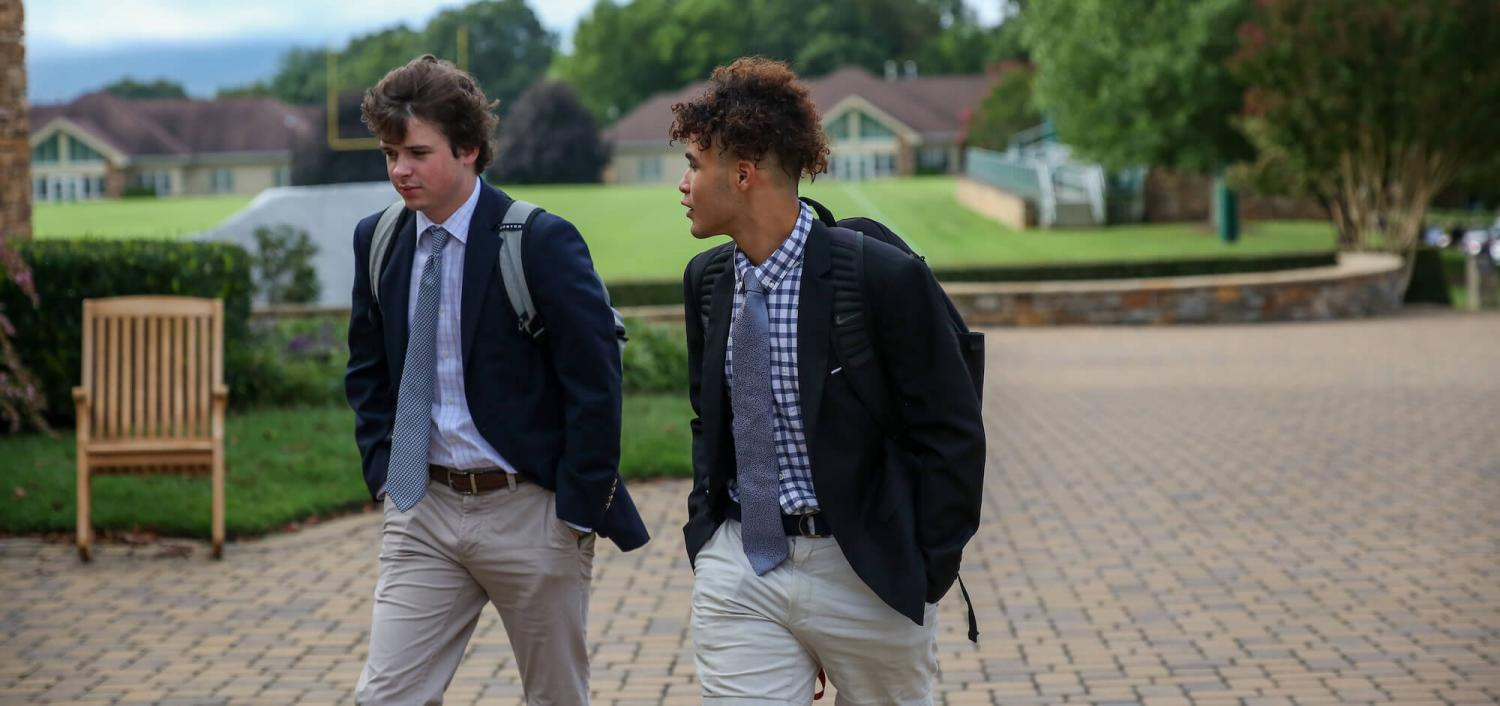# Mathematics

We believe the core of a strong mathematics curriculum stems from students being able to accomplish the following 5 principles:

### Problem Solving:

• Build new mathematical knowledge through problem solving
• Solve problems in mathematics and other disciplines.
• Learn a wide variety of problem solving techniques. Students can reflect on the process

### Reasoning:

• Recognize reasoning as a fundamental aspect of mathematics
• Make and investigate mathematical conjectures
• Develop and evaluate mathematical arguments and proofs

### Communication:

• Communicate their mathematical thinking coherently to peers, teachers, and others
• Use the language of mathematics to express ideas precisely
• Analyze and evaluate the mathematical thinking and strategy of others

### Connections:

• Understand how mathematical ideas interconnect and build on one another to produce a content whole.
• Recognize and can apply mathematics in contexts outside of mathematics.

### Representation

• Create and use representations to organize, record, and communicate mathematical ideas
• Select, apply, and translate among mathematical representations to solve problems
• Use representations to model and interpret physical, social, and mathematical phenomena

### CURRICULUM

All Christ School students are required to complete 4 years of mathematics. The core offerings of the mathematics department that all students complete are:

• Algebra I (regular or honors)
• Geometry (regular or honors)
• Algebra II (regular or honors)

After completing this core, students continue their mathematics studies by selecting at least 1 of the following.

• PreCalculus (regular or honors)
• Discrete Mathematics
• Calculus (pre-req Precalculus)
• AP Statistics  (pre-req - Algebra II)
• AP Calculus AB (pre-req Precalculus)
• AP Calculus BC (pre-req Precalculus)

We also offer the following semester courses for students who wish to further their mathematical studies beyond the typical high school curriculum:

• Differential Equations
• Linear Algebra
• Multivariable Calculus
• Graph Theory

In addition, we also offer the following elective courses in Computer Science

• AP Computer Science Principles
• AP Computer Science

### COMPETITIONS

Christ School’s mathematics department also encourages students to participate in mathematics and computer science competitions. The National High School Modeling Contest is offered in the Fall and the AMC is offered in the Spring for students each year.

#### Awards Won By Students:

2019: UNCA Programming contest - 7th place

2018: UNCA Programming contest - 7th place

2017: Honorable Mention National High School Modeling Contest
Honorable Mention National High School Modeling Contest
8th place UNCA Programming competition

2016: Meritorious award National High School Modeling Contest
Honorable Mention: International High School Modeling Contest

2015: AIMEE qualifier: Jerry Lu